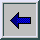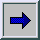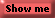#6. Calculate a ModelCalculating a model uses the transaction tables in your input directory, applies assignment rules in the model to make calculations, and stores the calculations in columns that are appended to your transaction tables in the output directory.

1. In any workspace, select a model.
2. Select ActionsCalculate Model.

The Calculate wizard opens.
3. Select the periods to calculate, or check Select all periods, and then click Next.

Note: Because each transaction table is associated with one and only one period, when you recalculate a model you do not need to recalculate all of the transaction tables. For example, you can calculate January and then February as a separate calculation. You never need to process a single period's transaction table more than once as the months proceed through the year.

4. Select the table groups to include in the calculation, and then click Next.
5. Select the cubes to generate, or check Select all reports, and then click Next.

Note: This window appears only if you have defined a summary report for this model.
6. Select the number of concurrent sessions to run.

If you are generating multiple table groups and multiple cubes, selecting multiple concurrent sessions can use parallel processing to speed up the calculation.
7. Select exception reports to generate and specify their suffix.
• A transaction exception report flags transaction rows that did not receive an assignment during the calculation.
• A behaviors exception report flags behaviors that were not assigned to any transaction during the calculation.

Note: If the name of the transaction table is long, the length of the suffix can result in the name of the exception report exceeding the maximum length of 32.
8. Specify the library to receive exception reports.

Note: If the library that you specify is the same as your output folder, then it can be difficult to distinguish the exception reports from the output tables in the output folder. Specifying a different library for the exception reports can make it easier to identify them.

9. Select Process limited data from the input transaction tables to validate model if you do not want a complete calculation; and specify the number of rows to calculate.

This option uncovers basic errors in your model without performing a full calculation.
10. Click Finish.
11. When the calculation finishes, click Details to view the message log.